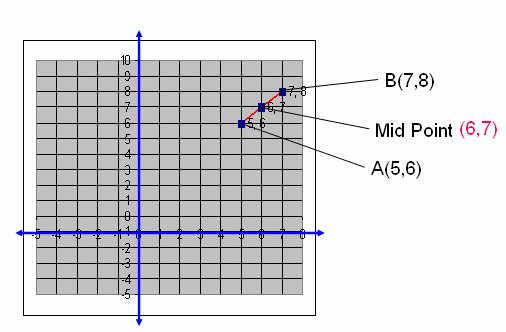Name: ___________________Date:___________________

kwizNET Subscribers, please login to turn off the Ads!
 Email us to get an instant 20% discount on highly effective K-12 Math & English kwizNET Programs!

### High School Mathematics - 29.8 Mid Point of the Segment Joining Two Points

 If A(x1 , y1) and B(x2 , y2) are two points, then the mid-point of the segment joining AB is [(x1 + x2)/2 , (y1 + y2)/2] i.e., (Sum of x-coordinates/2 , Sum of y-coordinates/2). Example: Find the mid point of (5,6) and (7,8). Solution: Formula for mid point of the segment joining two points is [(x1 + x2)/2 , (y1 + y2)/2] = [(5 + 7)/2 , (6 + 8)/2] = (12/2 , 14/2) = (6,7) Therefore, the mid point of (5,6) and (7,8) is (6,7). Showing the above problem in the Graph as below.Directions: Find the mid point of the segment joining two points and draw a graph each of the following questions. Also write at least 5 examples of your own.Name: ___________________Date:___________________

### High School Mathematics - 29.8 Mid Point of the Segment Joining Two Points

 Q 1: Find the mid point of the segment joining the points (3,4) and (7,8).(4,6)(5,6)(6,4)(6,5) Q 2: Find the mid point of the segment joining the points (3,4) and (4,5).(3.5,4.5)(4,5)(4.5,5.5)(3,4) Q 3: Find the mid point of the segment joining the points (7,8) and (11,12).(9,8)(10,9)(8,9)(9,10) Q 4: Find the mid point of the segment joining the points (4,8) and (2,4).(3,6)(6,3)(4,2)(2,4) Question 5: This question is available to subscribers only! Question 6: This question is available to subscribers only!

#### Subscription to kwizNET Learning System costs less than \$1 per month & offers the following benefits:

• Unrestricted access to grade appropriate lessons, quizzes, & printable worksheets
• Instant scoring of online quizzes
• Progress tracking and award certificates to keep your student motivated
• Unlimited practice with auto-generated 'WIZ MATH' quizzes
• Child-friendly website with no advertisements

© 2003-2007 kwizNET Learning System LLC. All rights reserved. This material may not be reproduced, displayed, modified or distributed without the express prior written permission of the copyright holder. For permission, contact info@kwizNET.com
For unlimited printable worksheets & more, go to http://www.kwizNET.com.